Let’s look at the airway dataset as an example of a typical small-scale RNA-Seq experiment. In this experiment, four Airway Smooth Muscle (ASM) cell lines are treated with the asthma medication dexamethasone.

The function weitrix_calibrate_all will be used to assign precision weights to log Counts Per Million values.

library(weitrix)
library(ComplexHeatmap)
library(EnsDb.Hsapiens.v86)
library(edgeR)
library(limma)
library(reshape2)
library(tidyverse)
library(airway)

set.seed(1234)

# BiocParallel supports multiple backends.
# If the default hangs or errors, try others.
# The most reliable backed is to use serial processing
BiocParallel::register( BiocParallel::SerialParam() )
data("airway")
airway
## class: RangedSummarizedExperiment
## dim: 64102 8
## assays(1): counts
## rownames(64102): ENSG00000000003 ENSG00000000005 ... LRG_98 LRG_99
## rowData names(0):
## colnames(8): SRR1039508 SRR1039509 ... SRR1039520 SRR1039521
## colData names(9): SampleName cell ... Sample BioSample

# Initial processing

Initial steps are the same as for a differential expression analysis.

counts <- assay(airway,"counts")

design <- model.matrix(~ dex + cell, data=colData(airway))

good <- filterByExpr(counts, design=design)
table(good)
## good
## FALSE  TRUE
## 47242 16860
airway_dgelist <- DGEList(counts[good,]) %>% calcNormFactors()

airway_lcpm <- cpm(airway_dgelist, log=TRUE, prior.count=1)

log2 CPM values have been calculated, with an added “prior” count of (on average) 1 read, so that zeros aren’t sent to negative infinity.

# Conversion to weitrix

airway_weitrix <- as_weitrix(airway_lcpm)

# Include row and column information
colData(airway_weitrix) <- colData(airway)
rowData(airway_weitrix) <-
mcols(genes(EnsDb.Hsapiens.v86))[rownames(airway_weitrix),c("gene_name","gene_biotype")]

airway_weitrix
## class: SummarizedExperiment
## dim: 16860 8
## assays(2): x weights
## rownames(16860): ENSG00000000003 ENSG00000000419 ... ENSG00000273487
##   ENSG00000273488
## rowData names(2): gene_name gene_biotype
## colnames(8): SRR1039508 SRR1039509 ... SRR1039520 SRR1039521
## colData names(9): SampleName cell ... Sample BioSample

# Calibration

To calibrate, we need predicted expression levels so we can calculate residuals. The function weitrix_components can provide linear model fits to each gene. (We will see a more advanced use of this function later.)

fit <- weitrix_components(airway_weitrix, design=design)

Currently the weitrix has weights uniformly equal to 1. Examining residuals, we see a variance trend relative to the linear model prediction.

Each dot in the plot below is a residual weighted by sqrt(weight). The x-axis is the linear model prediction. The y-axis is the weighted residual (all weights are currently 1). The red lines show the mean and the mean +/- one standard deviation.

weitrix_calplot(airway_weitrix, fit, covar=mu, guides=FALSE)We will use the function weitrix_calibrate_all to set the weights by fitting a gamma GLM with log link function to the weighted squared residuals. 1 over the predictions from this GLM are used as weights. Here we fit a natural spline based on the linear model predictions from fit, which are referred to in the formula as mu. well_knotted_spline is a wrapper around splines::ns for natural splines with improved choice of knot locations.

airway_cal <- weitrix_calibrate_all(
airway_weitrix,
design = fit,
trend_formula = ~well_knotted_spline(mu,5))
## mu range -5.552401 14.303375 knots -0.8637096  1.4763752  3.6914433  5.6106759  8.0243479
metadata(airway_cal)
## $weitrix ##$weitrix$x_name ##  "x" ## ##$weitrix$weights_name ##  "weights" ## ##$weitrix$all_coef ## (Intercept) well_knotted_spline(mu, 5)1 ## -0.4307917 -3.0128598 ## well_knotted_spline(mu, 5)2 well_knotted_spline(mu, 5)3 ## -4.0044737 -4.6128293 ## well_knotted_spline(mu, 5)4 well_knotted_spline(mu, 5)5 ## -3.3897229 -5.2701723 ## well_knotted_spline(mu, 5)6 ## -3.1086780 weitrix_calplot(airway_cal, fit, covar=mu)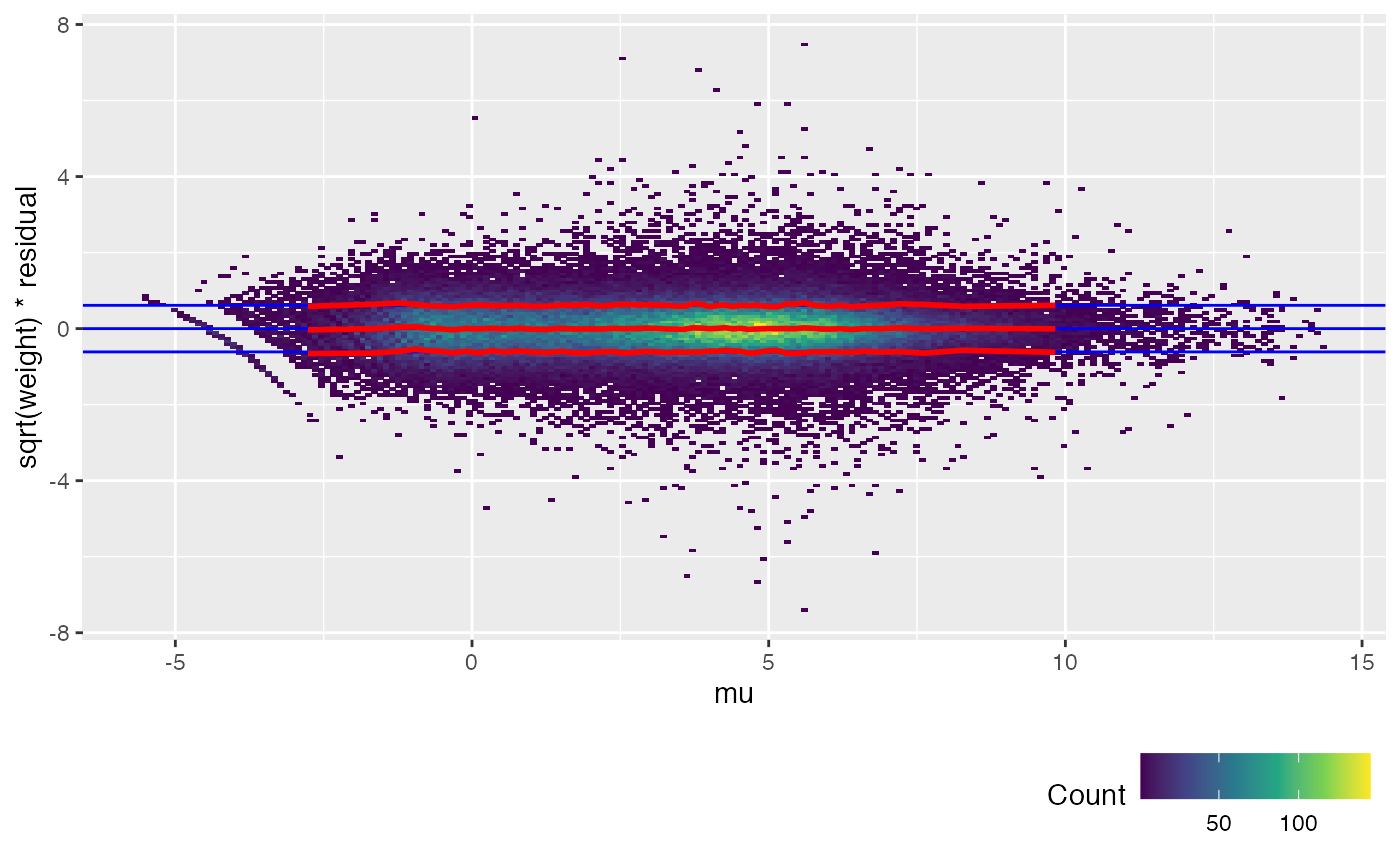The trend lines (red) for the calibrated weitrix are now uniformly close to 1, 0, -1 (guide lines shown in blue). Weights can now be treated as inverse residual variance. Another way to check this is to plot the unweighted residuals against 1/sqrt(weight), i.e. the residual standard deviation. weitrix_calplot(airway_cal, fit, funnel=TRUE)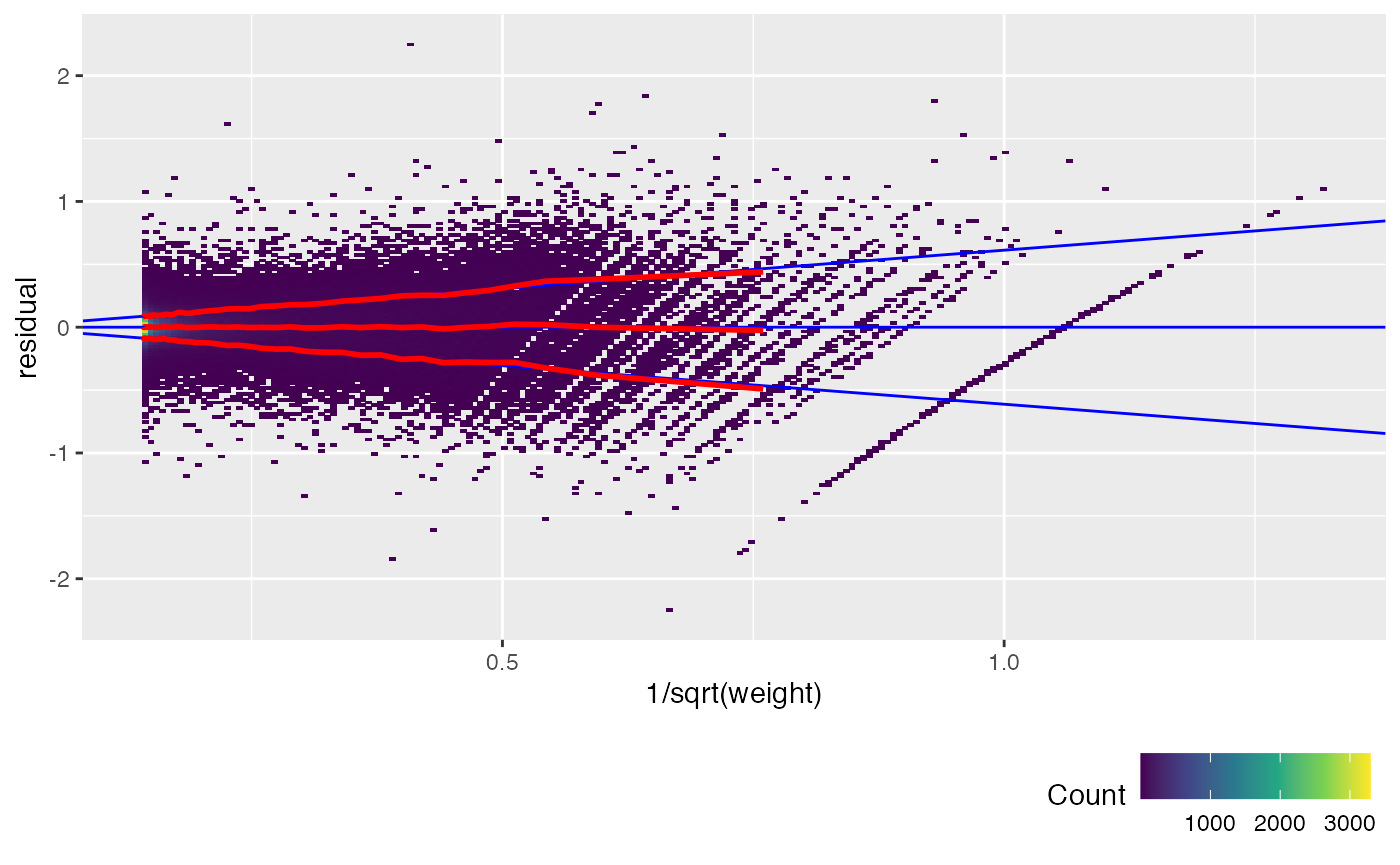We can also check each sample individually. weitrix_calplot(airway_cal, fit, covar=mu, cat=col)## Advanced calibration If there was an odd sample, we could use a more complex trend formula such as ~col*well_knotted_spline(mu,4): airway_cal <- weitrix_calibrate_all( airway_weitrix, design = fit, trend_formula = ~col*well_knotted_spline(mu,4)) ## Similar to limma voom While the precise details differ, what we have done is very similar to the voom function in limma. weitrix_calplot can be used with voomed data as well. airway_voomed <- voom(airway_dgelist, design, plot=TRUE)weitrix_calplot(airway_voomed, design, covar=mu)voom achieves a similar result to weitrix_calibrate_all (but note the input to voom is a DGEList of counts, not a matrix that is already log transformed). limma EList objects can be converted to weitrix objects with as_weitrix. Weitrix objects can be converted to limma EList objects with weitrix_elist. # Exploration RNA-Seq expression is well trodden ground. The main contribution of the weitrix package here is to aid exploration by discovering components of variation, providing not just column scores but row loadings and respecting precision weights. ## Find genes with excess variation weitrix_sd_confects will find genes with excess variation in a calibrated weitrix. confects <- weitrix_sd_confects(airway_cal) confects ##$table
##    confect effect row_mean typical_obs_err dispersion n_present df fdr_zero
## 1  2.620   3.121  6.631    0.2930          114.49     8         7  0
## 2  2.608   3.020  4.731    0.2495          147.49     8         7  0
## 3  2.368   2.785  3.850    0.2573          118.16     8         7  0
## 4  2.076   2.484  4.006    0.2557           95.41     8         7  0
## 5  2.009   2.271  8.249    0.1658          188.60     8         7  0
## 6  1.993   2.477  2.849    0.3102           64.79     8         7  0
## 7  1.905   2.208  4.396    0.1961          127.83     8         7  0
## 8  1.893   2.334  3.054    0.2875           66.93     8         7  0
## 9  1.892   2.145  6.065    0.1657          168.64     8         7  0
## 10 1.856   2.114  5.431    0.1702          155.23     8         7  0
##    name            gene_name gene_biotype
## 1  ENSG00000229807 XIST      lincRNA
## 2  ENSG00000262902 MTCO1P40  processed_pseudogene
## 3  ENSG00000171819 ANGPTL7   protein_coding
## 4  ENSG00000243137 PSG4      protein_coding
## 5  ENSG00000123243 ITIH5     protein_coding
## 6  ENSG00000198732 SMOC1     protein_coding
## 7  ENSG00000162817 C1orf115  protein_coding
## 8  ENSG00000235750 KIAA0040  protein_coding
## 9  ENSG00000096060 FKBP5     protein_coding
## 10 ENSG00000204941 PSG5      protein_coding
## ...
## 6216 of 16860 non-zero excess standard deviation at FDR 0.05

“effect” is root-mean-square variation in residuals relative to a fitted model in excess of what is expected from the calibrated weights. Here the model only has an intercept term, so the residuals represent variation from the weighted mean. “confect” scores are lower confidence bounds on the effect, adjusted for multiple testing using the topconfects method.

In single-cell experiments, this should be a good way to find marker genes.

Top genes can then be examined to find a reason for their variation. For example, we see that XIST is highly expressed in a particular cell type.

interesting <- confects$table$index[1:20]

centered <- weitrix_x(airway_cal) - rowMeans(weitrix_x(airway_cal))
rownames(centered) <- rowData(airway_cal)$gene_name Heatmap( centered[interesting,], name="log2 RPM\nvs row mean", cluster_columns=FALSE)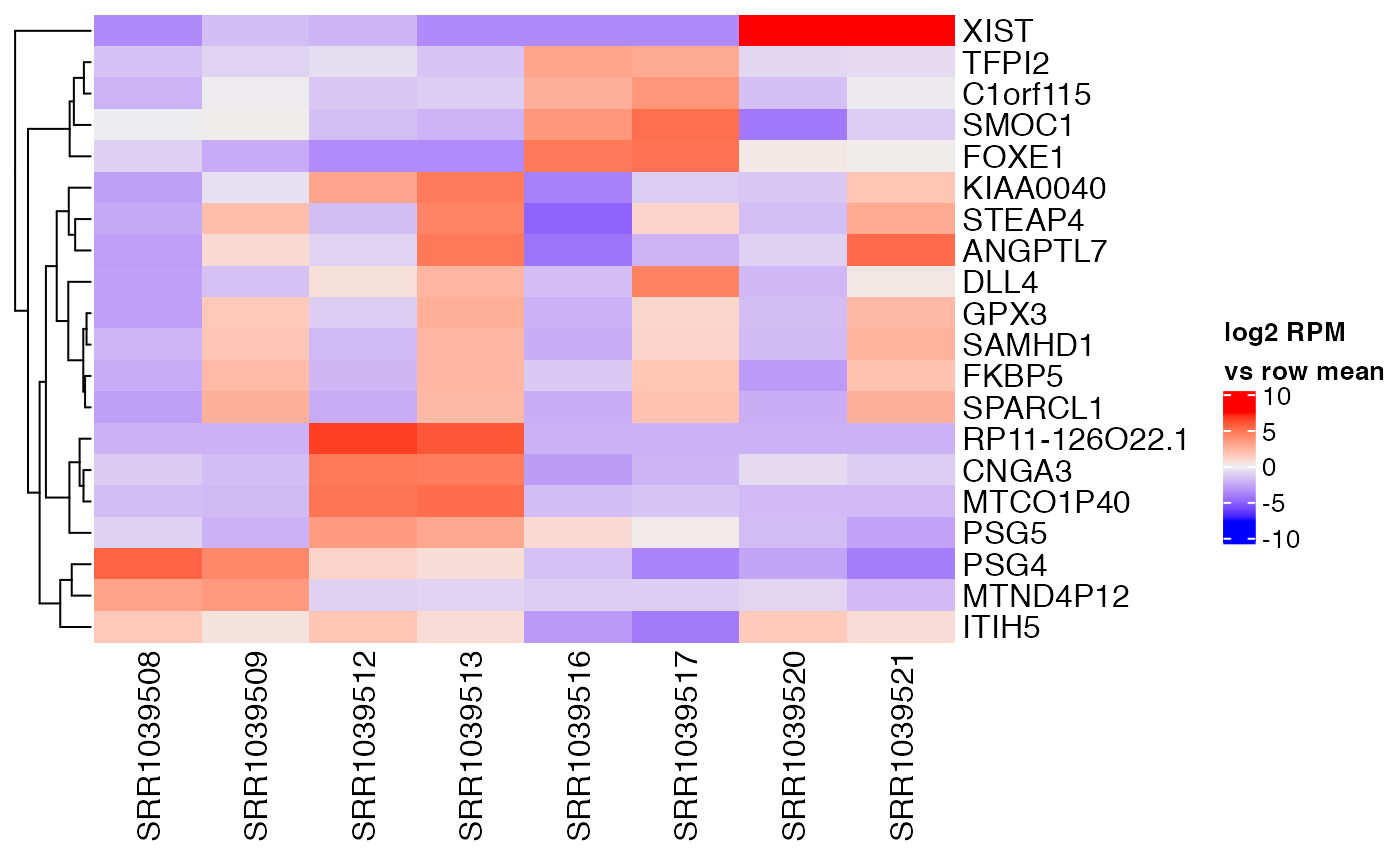## Find components of variation The code below will find various numbers of components, from 1 to 6. In each case, the components discovered have varimax rotation applied to their gene loadings to aid interpretability. The result is a list of Components objects. comp_seq <- weitrix_components_seq(airway_cal, p=6, n_restarts=1) comp_seq ## [] ## Components are: (Intercept), C1 ##$row :  16860 x 2 matrix
## $col : 8 x 2 matrix ##$R2  :  0.4046082
##
## []
## Components are: (Intercept), C1, C2
## $row : 16860 x 3 matrix ##$col :  8 x 3 matrix
## $R2 : 0.6364208 ## ## [] ## Components are: (Intercept), C1, C2, C3 ##$row :  16860 x 4 matrix
## $col : 8 x 4 matrix ##$R2  :  0.8055695
##
## []
## Components are: (Intercept), C1, C2, C3, C4
## $row : 16860 x 5 matrix ##$col :  8 x 5 matrix
## $R2 : 0.9096617 ## ## [] ## Components are: (Intercept), C1, C2, C3, C4, C5 ##$row :  16860 x 6 matrix
## $col : 8 x 6 matrix ##$R2  :  0.9500206
##
## []
## Components are: (Intercept), C1, C2, C3, C4, C5, C6
## $row : 16860 x 7 matrix ##$col :  8 x 7 matrix
## $R2 : 0.9778973 We can compare the proportion of variation explained to what would be explained in a completely random weitrix. Random normally distributed values are generated with variances equal to one over the weights. rand_weitrix <- weitrix_randomize(airway_cal) rand_comp <- weitrix_components(rand_weitrix, p=1, n_restarts=1) components_seq_screeplot(comp_seq, rand_comp)## Examining components Up to 4 components may be justified. comp <- comp_seq[] comp$col[,-1] %>% melt(varnames=c("Run","component")) %>%
left_join(as.data.frame(colData(airway)), by="Run") %>%
ggplot(aes(y=cell, x=value, color=dex)) +
geom_vline(xintercept=0) +
geom_point(alpha=0.5, size=3) +
facet_grid(~ component) +
labs(title="Sample scores for each component", x="Sample score", y="Cell line", color="Treatment")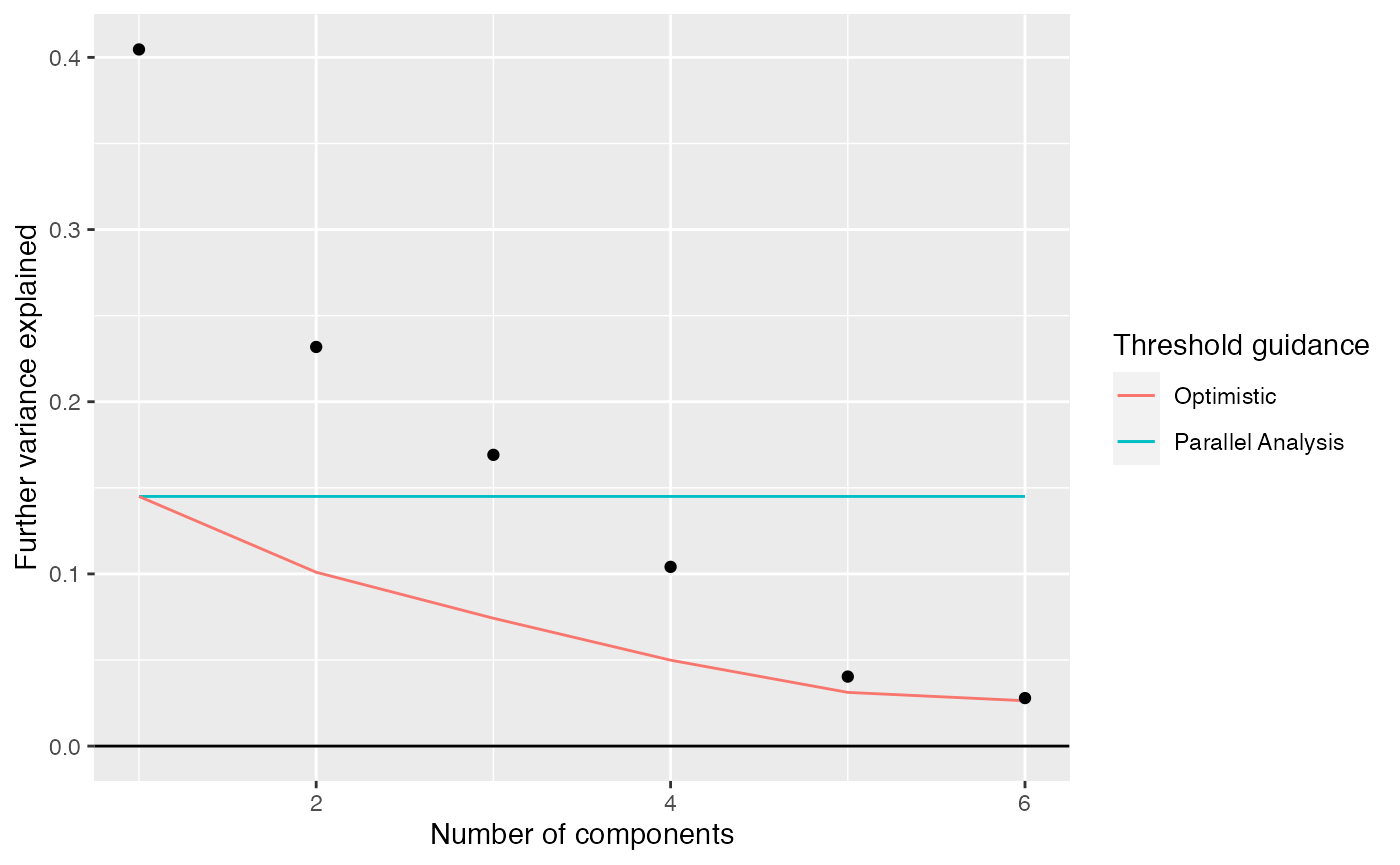comp$row[,-1] %>% melt(varnames=c("name","component")) %>% ggplot(aes(x=comp$row[name,"(Intercept)"], y=value)) +
geom_point(cex=0.5, alpha=0.5) +
facet_wrap(~ component) +
labs(title="Gene loadings for each component vs average log2 RPM", x="average log2 RPM", y="gene loading")## Without varimax rotation, components may be harder to interpret

If varimax rotation isn’t used, weitrix_components and weitrix_components_seq will produce a Principal Components Analysis, with components ordered from most to least variance explained.

Without varimax rotation the treatment effect is still mostly in the first component, but has also leaked a small amount into the other components.

comp_nonvarimax <- weitrix_components(airway_cal, p=4, use_varimax=FALSE)

comp_nonvarimax$col[,-1] %>% melt(varnames=c("Run","component")) %>% left_join(as.data.frame(colData(airway)), by="Run") %>% ggplot(aes(y=cell, x=value, color=dex)) + geom_vline(xintercept=0) + geom_point(alpha=0.5, size=3) + facet_grid(~ component) + labs(title="Sample scores for each component, no varimax rotation", x="Sample score", y="Cell line", color="Treatment")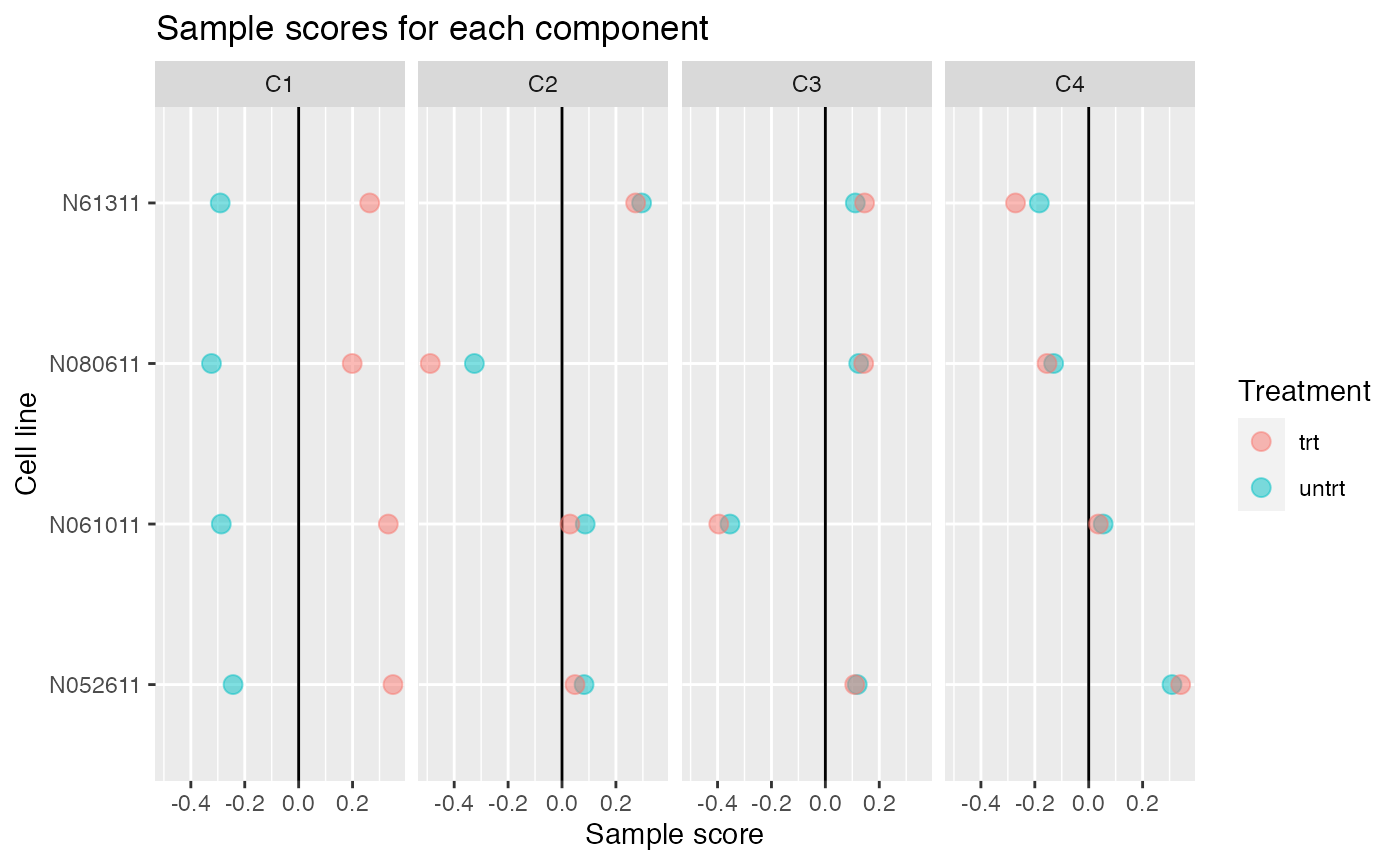## col can potentially be used as a design matrix If you’re not sure of the experimental design, for example the exact timing of a time series or how evenly a drug treatment was applied, the extracted component might actually be more accurate. Note that this ignores uncertainty about the col matrix itself. This may be useful for hypothesis generation – finding some potentially interesting genes, while discounting noisy or lowly expressed genes – but don’t use it as proof of significance. First by the topconfects method. This will find the largest confident effect sizes, while still correcting for multiple testing. weitrix_confects(airway_cal, comp$col, "C1")
## $table ## confect effect se df fdr_zero row_mean typical_obs_err ## 1 6.606 12.075 0.5919 9.195 2.653e-06 2.311 0.2012 ## 2 6.463 11.783 0.6258 9.195 3.736e-06 4.755 0.2381 ## 3 5.997 7.957 0.2423 9.195 3.073e-07 4.746 0.1522 ## 4 5.956 9.320 0.4307 9.195 1.907e-06 3.850 0.2540 ## 5 5.746 7.873 0.2799 9.195 4.763e-07 5.405 0.1935 ## 6 5.510 6.586 0.1466 9.195 7.303e-08 7.758 0.1171 ## 7 5.510 8.310 0.3843 9.195 1.907e-06 3.399 0.2057 ## 8 5.236 8.871 0.5074 9.195 5.201e-06 4.021 0.2664 ## 9 5.069 9.497 0.6276 9.195 1.135e-05 2.014 0.2745 ## 10 -5.062 -6.453 0.1999 9.195 3.073e-07 4.648 0.1340 ## name gene_name gene_biotype ## 1 ENSG00000179593 ALOX15B protein_coding ## 2 ENSG00000109906 ZBTB16 protein_coding ## 3 ENSG00000163884 KLF15 protein_coding ## 4 ENSG00000171819 ANGPTL7 protein_coding ## 5 ENSG00000152583 SPARCL1 protein_coding ## 6 ENSG00000101347 SAMHD1 protein_coding ## 7 ENSG00000168309 FAM107A protein_coding ## 8 ENSG00000127954 STEAP4 protein_coding ## 9 ENSG00000250978 RP11-357D18.1 processed_transcript ## 10 ENSG00000162692 VCAM1 protein_coding ## ... ## 5540 of 16860 non-zero contrast at FDR 0.05 ## Prior df 6.2 If you prefer limma and p-values: airway_elist <- weitrix_elist(airway_cal) fit <- lmFit(airway_elist, comp$col) %>%
eBayes()

fit$df.prior ##  6.19524 fit$s2.prior
##  0.5946888
topTable(fit, "C1")
##                 gene_name   gene_biotype     logFC  AveExpr         t
## ENSG00000101347    SAMHD1 protein_coding  6.585680 8.135498  44.90834
## ENSG00000189221      MAOA protein_coding  5.730034 5.951528  34.04168
## ENSG00000178695    KCTD12 protein_coding -4.417195 6.452192 -31.72330
## ENSG00000139132      FGD4 protein_coding  3.809042 5.416374  31.54337
## ENSG00000179094      PER1 protein_coding  5.479478 4.423081  30.43529
## ENSG00000120129     DUSP1 protein_coding  5.120892 6.644985  29.38798
## ENSG00000162692     VCAM1 protein_coding -6.453409 3.576221 -32.29109
## ENSG00000124766      SOX4 protein_coding -4.273940 5.427907 -28.70344
## ENSG00000163884     KLF15 protein_coding  7.957297 3.249262  32.84342
## ENSG00000165995    CACNB2 protein_coding  5.717502 3.686989  29.63384
## ENSG00000101347 4.331667e-12 7.303191e-08 17.48757
## ENSG00000189221 5.456200e-11 3.073475e-07 15.61359
## ENSG00000178695 1.038475e-10 3.073475e-07 15.14447
## ENSG00000139132 1.093763e-10 3.073475e-07 15.08271
## ENSG00000179094 1.515295e-10 3.649695e-07 14.56555
## ENSG00000120129 2.084677e-10 3.905294e-07 14.51588
## ENSG00000162692 8.833396e-11 3.073475e-07 14.50721
## ENSG00000124766 2.583657e-10 4.356046e-07 14.30694
## ENSG00000163884 7.567087e-11 3.073475e-07 14.29881
## ENSG00000165995 1.932349e-10 3.905294e-07 14.13861
all_top <- topTable(fit, "C1", n=Inf, sort.by="none")
plotMD(fit, "C1", status=all_top\$adj.P.Val <= 0.01)